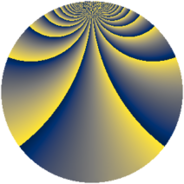# Properties

 Label 3549.2.coLevel $3549$ Weight $2$ Character orbit 3549.co Rep. character $\chi_{3549}(64,\cdot)$ Character field $\Q(\zeta_{26})$ Dimension $2208$ Sturm bound $970$

# Related objects

## Defining parameters

 Level: $$N$$ $$=$$ $$3549 = 3 \cdot 7 \cdot 13^{2}$$ Weight: $$k$$ $$=$$ $$2$$ Character orbit: $$[\chi]$$ $$=$$ 3549.co (of order $$26$$ and degree $$12$$) Character conductor: $$\operatorname{cond}(\chi)$$ $$=$$ $$169$$ Character field: $$\Q(\zeta_{26})$$ Sturm bound: $$970$$

## Dimensions

The following table gives the dimensions of various subspaces of $$M_{2}(3549, [\chi])$$.

Total New Old
Modular forms 5856 2208 3648
Cusp forms 5760 2208 3552
Eisenstein series 96 0 96

## Trace form

 $$2208q + 188q^{4} - 184q^{9} + O(q^{10})$$ $$2208q + 188q^{4} - 184q^{9} + 4q^{13} - 196q^{16} - 16q^{17} + 136q^{22} + 8q^{23} + 224q^{25} - 20q^{26} + 8q^{29} + 8q^{30} + 104q^{31} + 260q^{34} + 188q^{36} - 56q^{38} - 8q^{39} - 40q^{40} - 4q^{42} + 32q^{48} + 184q^{49} + 24q^{51} - 280q^{52} - 28q^{53} - 172q^{55} + 52q^{58} - 208q^{60} + 8q^{61} - 180q^{62} + 188q^{64} - 28q^{65} + 192q^{66} + 52q^{67} + 16q^{68} - 8q^{69} - 16q^{74} + 208q^{76} + 16q^{77} - 224q^{78} - 8q^{79} - 184q^{81} - 220q^{82} + 52q^{85} + 176q^{87} + 520q^{88} + 4q^{91} - 56q^{92} + 152q^{94} + 192q^{95} + O(q^{100})$$

## Decomposition of $$S_{2}^{\mathrm{new}}(3549, [\chi])$$ into newform subspaces

The newforms in this space have not yet been added to the LMFDB.

## Decomposition of $$S_{2}^{\mathrm{old}}(3549, [\chi])$$ into lower level spaces

$$S_{2}^{\mathrm{old}}(3549, [\chi]) \cong$$ $$S_{2}^{\mathrm{new}}(169, [\chi])$$$$^{\oplus 4}$$$$\oplus$$$$S_{2}^{\mathrm{new}}(507, [\chi])$$$$^{\oplus 2}$$$$\oplus$$$$S_{2}^{\mathrm{new}}(1183, [\chi])$$$$^{\oplus 2}$$